# Fund Characteristics

This section describes the set of mutual funds used for the analyses that follow. It also provides information on key characteristics of such funds. Expenses, turnover ratios and total assets for some funds as of December 1993 were taken from the Principia Plus CD-ROM issued in January 1994. All other data were obtained from Morningstar's Principia Plus CD-ROM issued in January 1997 which included data on fund returns and characteristics through the end of December 1996.

### Funds and Total Assets

The following table shows the number of funds as of December 1996 for which Morningstar data were available for the last 1,3,5 and 10 years:

Number of funds, December 1996

 Asset Class 1 Year 3 Years 5 Years 10 Years Domestic Equity 2,959 1,826 1,058 598 International Equity 835 383 185 60 Taxable Bond 1,670 1,104 597 242 Municipal Bond 1,739 1,129 580 257 Total 7,203 4,442 2,430 1,157

Our analyses focus on funds with at least three years of data. The table below shows the number of such funds in each asset class, their total assets at the end of 1996, and the average amount of assets per fund.

Number of funds, assets (\$ million), and assets/fund (\$ million), December 1996

 Funds Assets Assets/Fund Domestic Equity 1,826 1,340,582 734 International Equity 383 230,570 602 Taxable Bond 1,104 339,307 307 Municipal Bond 1,129 243,966 216 Total 4,442 2,154,425 485

#### The 3-year Sample of Diversified Equity Funds

As the previous table shows, domestic equity funds have well over half the assets. Moreover, the bulk of those assets are held by funds which Morningstar assigns to its nine diversified equity categories. At the end of 1996, there were 1,306 such funds. Of these, 18 were not assigned to categories and were excluded from this study since our goal is to compare all the performance measures including those utilizing category information. In addition, two of the funds had extremely high expense ratios (greater than 10%). They were also excluded in order to avoid outliers that could dominate both performance statistics and some of the regression analyses.

The tables below show, respectively, the number of funds, total assets at the end of 1996, and average assets per fund for the1,286 remaining funds:

Number of funds, December 1996

 Value Blend Growth Total Large 159 370 107 636 Medium 79 123 170 372 Small 94 74 110 278 Total 332 567 387 1,286

Assets (\$ million), December 1996

 Value Blend Growth Total Large 185,093 349,939 105,759 640,790 Medium 44,989 149,009 136,966 330,963 Small 28,207 19,171 49,557 96,934 Total 258,287 518,118 292,282 1,068,687

Assets/Fund (\$ million), December 1996

 Value Blend Growth Total Large 1,164 946 988 1,008 Medium 570 1,212 806 890 Small 300 259 451 349 Total 778 914 755 831

As can be seen, blend funds held more assets than either value or growth funds. Large-capitalization funds held considerably more assets than medium-capitalization funds, which in turn held considerably more assets than small-capitalization funds. Finally, small-capitalization funds were themselves small, holding fewer assets per fund on average than their medium and large-capitalization counterparts.

These 1,286 diversified equity funds make up our main ("3-year") sample. At the end of 1996, they held approximately half the assets held by mutual funds with at least three years of history, and thus constituted a major portion of the industry.

#### The 6-year Sample of Diversified Equity Funds

For some analyses we utilize the subset of the funds in the 3-year sample for which returns are available beginning with December 1990 or earlier and for which expense ratios, turnover percentages and total assets as of the end of 1993 were included on the Morningstar CDROM produced at the time. Of the 1,286 funds in the 3-year sample, 541 fulfilled these conditions. The primary reason that this is a much smaller sample is the rapid increase in the number of funds in the 1990's. Many of the funds in existence in 1994 were formed during the prior 3 years. However, some of the funds in existence at the end of 1993 undoubtedly subsequently disappeared through merger or liquidation or changed objective sufficiently to be renamed and/or reclassified by Morningstar. For the latter reasons, the 6-year sample is subject to survivor biases of unkown magnitude and results of our "out of sample" tests should be interepreted with considerable caution.

#### Expense Ratios

Two characteristics that affect the net performance of a fund are (1) the costs of management and administration, summarized in its expense ratio and (2) the magnitude of its security purchases and sales, summarized in its turnover ratio.

Morningstar's expense ratio is:

"A figure taken from the fund's prospectus that represents the percentage of fund assets paid for operating expenses and management fees, including 12b-1 fees, administrative fees, and all other asset-based costs incurred by the fund, except brokerage costs.... Sales charges are not included.." 1

The following diagrams show the cumulative distribution of expense ratios. For convenience, only ratios from 0 to 2% are shown, although the entire range is used for calculations and the determination of the curves.

In the first case, each fund is given equal weight. The vertical axis thus shows the proportion of funds with expense ratios smaller than the value shown at the corresponding point on the horizontal axis. For example, to see the median expense ratio, select the 0.5 point on the vertical axis. Move right to the left-most point on of the shaded distribution, then down to the horizontal axis. The value on the latter is 1.19. Thus, 50% (0.50) of the funds had expense ratios smaller than 1.19 (and 50% had ratios greater than 1.19). Continuing in this manner, the graph shows that 20% (0.20 on the vertical axis) of the funds had expense ratios smaller than approximately 0.90 (on the horizontal axis), and 80% thus had ratios greater than 0.90.

Cumulative distribution of expense ratios: equally-weighted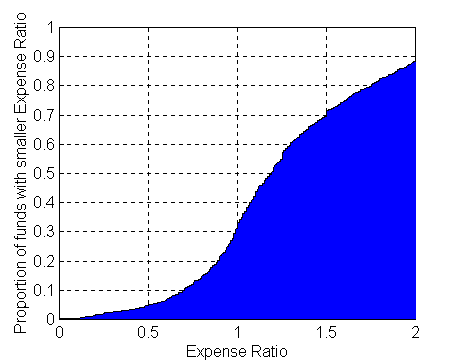While it is interesting to determine the expense ratio for the average fund, for many purposes it is more relevant to determine the expense ratio for the average dollar invested in a set of mutual funds. To do so, one must weight larger funds more heavily than smaller funds. The diagram below does this. As can be seen, the median expense ratio is considerably smaller (well less than 1.00).

Cumulative distribution of expense ratios: dollar-weighted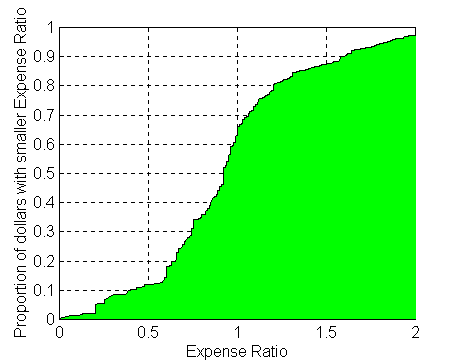The table below provides summary information for both cases. Due to the presence of a few funds with very high expense ratios (not shown on the graphs), the mean is higher than the median in each case. Moreover, the values are considerably lower when dollar weights are used instead of equal weights. Perhaps the most relevant number in the table is the one in the lower left. Investors in this group of mutual funds collectively paid expenses equal to 0.952%, or 95.2 cents per year on every \$100 invested. It is reassuring that the dollar-weighted figures are considerably smaller than the frequently quoted and less relevant equal-weighted numbers (such as the 1.305% in this sample). Note, however, that the inclusion of low-expense index funds in the sample gives lower values than would be obtained if only actively-managed funds were analyzed.

Expense ratios

 Mean Median Equal-Weighted 1.305 1.190 Dollar-Weighted 0.952 0.920

#### Turnover Ratios

Morningstar obtains the turnover ratio from fund shareholder reports. To compute it,

"A fund divides the lesser of purchases or sales (expressed in dollars and excluding all securities with maturities of less than one year) by the fund's average monthly assets." 2

The diagrams and table below provide information on the turnover ratios of the funds in our sample. Only ratios from 0 to 200 percent are shown, although all values are used for the calculations and the determination of the curves.

Cumulative distribution of turnover ratios: equal-weighted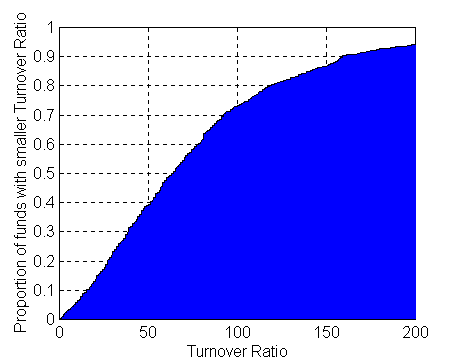Cumulative distribution of turnover ratios: dollar-weighted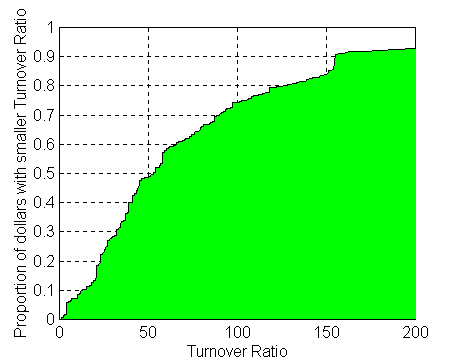Turnover ratios

 Mean Median Equal-Weighted 80.4 64.0 Dollar-Weighted 73.8 53.0

Here, too, the dollar-weighted mean is the most relevant. Approximately 73.8% of the dollars invested in this set of diversified equity funds were turned over each year. This clearly added to fund and investor's costs. Whether or not commensurate gains in value were obtained remains to be seen.

#### Relationships among Fund Characteristics

The difference between the dollar-weighted and equal-weighted expense ratios shows that on average, larger funds have lower expense ratios due, no doubt, to their economies of scale and ability to spread fixed costs over more assets. The diagram below shows that this holds quite regularly. Each bar shows the (equally-weighted) average expense ratio of the funds in each of ten deciles (129 fundsin each of the first nine deciles and 125 in the tenth) based on assets under management. Thus the average fund in the lowest size decile charged 1.89% per year in expenses, while the average fund in the largest size decile charged 0.96%.

Average expense ratios for ten deciles based on asset value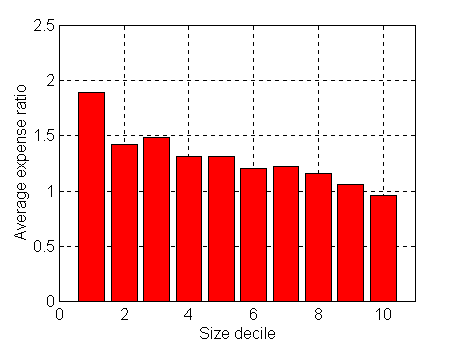Size does not appear to be closely related to turnover as the diagram below indicates.

Average turnover ratios for ten deciles based on asset value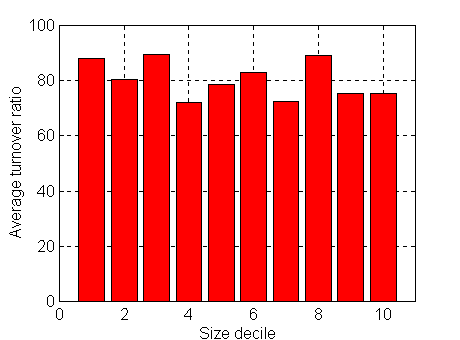While size is not related to turnover, expense ratio is, as the diagram below shows. The roughly ten percent of funds with the lowest expense ratios averaged a turnover of slightly over 40%, while those with the highest expense ratios averaged more than 100%. Since the costs of trading (explicit and implicit) are generally excluded when computing expense ratios, this suggests that funds with high stated expenses tend to also have high unstated expenses. Since both types of expenses reduce returns, managers of such funds would have to increase gross returns by larger amounts than their low-expense counterparts in order to even match the latter's net returns. Analyses of net performance are required to see if they succeed.

Average turnover ratios for ten deciles based on expense ratios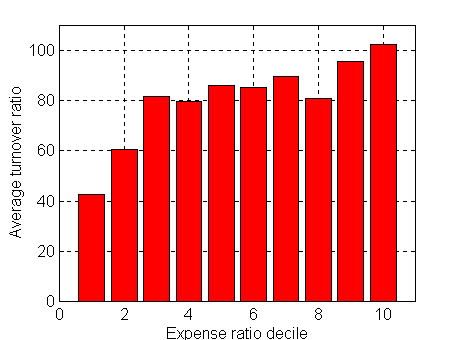1. Advanced Analytics for Morningstar Principia Plus

2. Morningstar Mutual Funds User's Guide